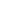| lesson material | material summary | questions and answers | definitions | types and examples | other information | materi pelajaran | ringkasan materi | pertanyaan dan jawaban | definisi | jenis-jenis dan contoh-contoh | informasi lainnya |

# Rules text entry algorithms, as well as the concept of data types, variables, constants and operators

Rules text entry algorithms, as well as the concept of data types, variables, constants and operators

In this occasion I will share the algorithms and programming that includes an introduction to the algorithm, the basics of algorithms, rules text entry algorithms, as well as the concept of data types, variables, constants and operators.

INTRODUCTION Algorithm
Basic concepts

"The necessity of each person who wants to resolving a problem of logic in a structured, effective, and efficient"
definitions algorithm

"The arrangement is a logical, systematic, and finite to memecahlkan a problem or to achieve certain goals"

BASICS Algorithm
The characteristics of the algorithm
· Algorithms have a beginning and an end,
· Each step must be defined properly, so it does not have a double meaning, not confusing (not ambiguous).
· Have the input (input) or initial conditions.
· Have output (output) or the final conditions.
· Algorithms must be effective, should be followed absolutely it will solve the problem.

RULES FOR WRITING TEXT Algorithm
1. Title

There should be no spaces,

There should be no special symbol except the symbol "_" underscroll.

Should interpretative (according to what the algorithm).
example:
Luas_Segitiga
{Algorithms calculate the area of ​​a triangle}

2. Dictionary (Declaration)

"As a place for the definition: data type name, constant name, variable name, function name, the name of the procedure."
example:
Declaration:
{Length = integer data type integer}
Width = integer
Size = real data type fractions {}

3. Algorithm (Description)

Text components in a procedural programming algorithm:

Basic instructions such as input / output and assignment.

Sequence (sequence)

analysis of cases

Looping (iteration)

example:
Description:
inputs (length, width)
spacious <- length * width
output (wide)

CONCEPT TYPE DATA, VARIABLES, CONSTANTS AND OPERATOR
Data types
The data type is the type of data that can be processed by computer to meet the needs in computer programming.
The data type is divided into three, namely:

simple type

String Type

Structured types
1. Type Simple
Divided into three, namely:

A. Numeric

1. Integer

2. Float, is divided into two:

a. Single

b. Double

B. Boolean

IF ... THEN

IF ... THEN ... ELSE
C. Character

2. Type String

A. String

3. Structured Type

A. Array

B. Record

C. Set

variable
A variable is a name that states place in the computer memory to store a value and its value may be changed at any time - when the program is executed.
Examples of variable names and values.
Name = "Al-Khoir"
Price = 3000
HargaTotal = 90000
username, name, price and HargaTotal is the name of a variable, while the "Doni", "Al-Khoir", 3000 and 90000 is the value of each variable.These values ​​will be stored in the variable name of each round does not change.
Giving the name of the variable must follow the rules set by the programming language that we use. But in general there is a rule that applies to almost all programming languages. The rules are:
· Can not use spaces in a variable name. Spaces can be replaced with the underscore character (_).
· The variable name should not contain special characters, such as:., +, -, *, /, <,>, &, (,) And others.
· The variable name can not use key words in a programming language.

constants
The constant is a variable whose data values ​​are fixed and can not be changed. So constant is also variable is the difference between the value of the deposit. If the value of the data along the walking program is not changed to change, then a better variables are treated as constants. In a program code, usually the data values ​​of the constants given directly at the constant declarations. As for the variables usually only defined variable names and data types without stuffing data values. Variable naming rule also applies to naming constants. Likewise, the determination rule data type. constant divided into four:
Integer constant, such as regular integer, long integer (long int) and unsigned integer (unsigned integer). Its value may be decimal, octal or hexadecimal.
Floating point constants, may contain a fractional value, which is usually written in the form of common fraction as well as the exponential form and is always expressed in a double, unless terminated by F or f (declared constants float).
The constant string, a sequence of characters that starts and ends with double quotation marks ( "..."). May also contain characters that use the \ called the escape character (escape sequence).
Character constants, always begins and ends with single quotes ( '...'). Some character constants can be preceded by a \ (promulgated after the single quotation marks).For example, if we make a mathematical calculation program that uses the value of pi (3.14159) which may appear in many places in the source code, we can make as a constant pi. The use of constant pi will further facilitate the writing program code rather than having to type in the value of 3.14159 repeatedly.

Operator1. Arithmetic
This operator is used for the operation aritmatika.Operand (magnitudes operated) can be variables, constants, or a nilai.Operand operated by this operator can be numeric, integer, or real.
2. Operator Relationships
Relation been used to compare the relationship between the two operands and we will get the results of boolean type, ie TRUE or FALSE.Operator relations, among others:
Data: A = 5
B = 8
C = 5

That's all I can post this time, may be useful. thanks.
##### 0 Komentar untuk "Rules text entry algorithms, as well as the concept of data types, variables, constants and operators"

Silahkan berkomentar sesuai artikel

Template By Kunci Dunia